# How to calculate average cells from different sheets in Excel?

How can we do the calculations needed to determine the average of all the numbers on the various worksheets if we want to accomplish that? This post will show you an easy approach to compute the average for all numbers across several worksheets. Currently, we may know how to calculate the average for a selected range on a single worksheet. However, this article will show you how to do it for all numbers.

In terms of the reality that the data range on other worksheets could be the same or different, we have prepared two instances to use as an illustration.

Let’s understand step by step with an example.

### Step 1

To begin, we will need to prepare two worksheets by populating them with numbers from the same range or different range. As shown in the below screenshots.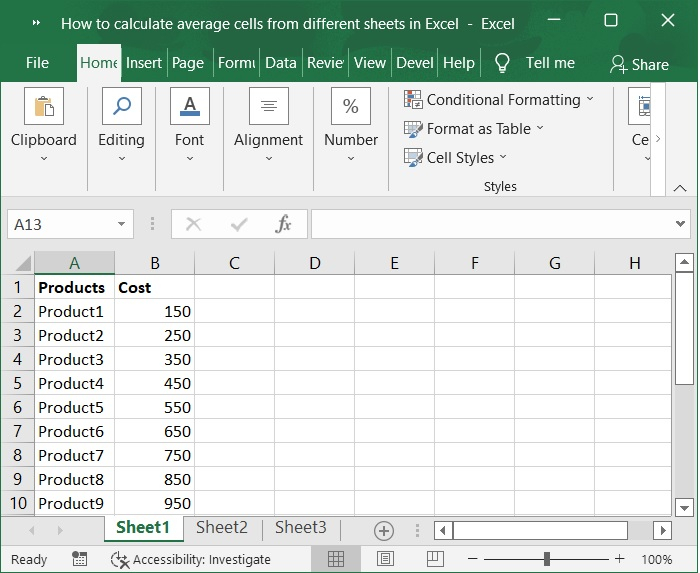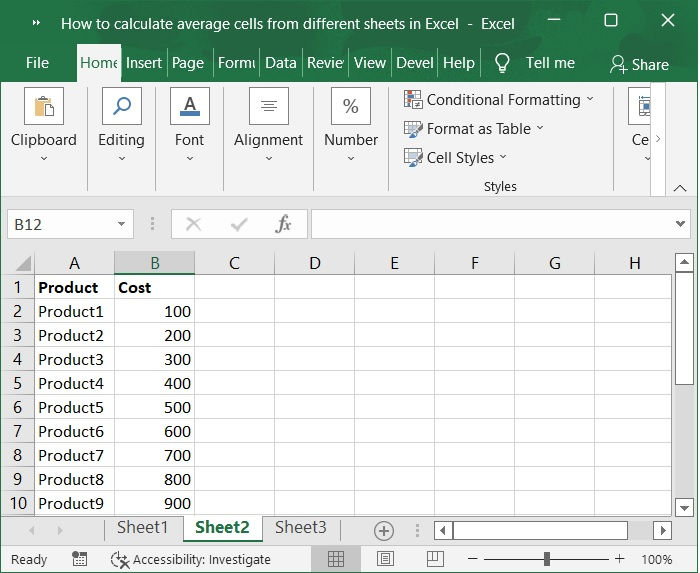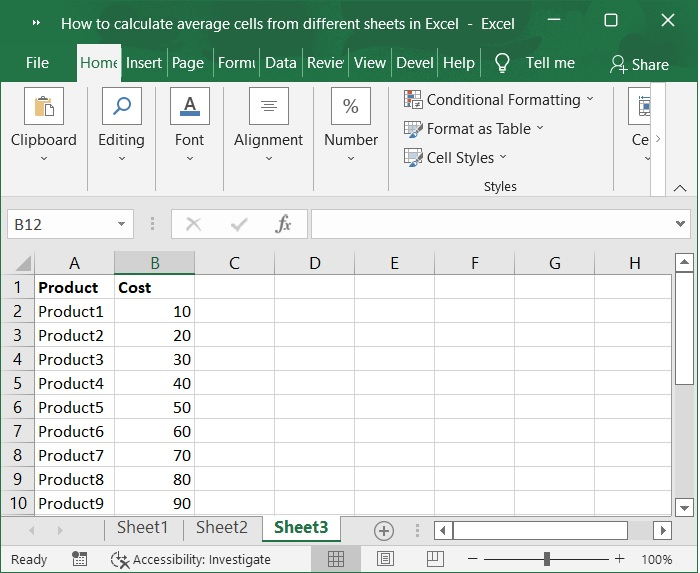If, for instance, you want to calculate the average of Range A1:A10 across Sheets 1 through 3, you may easily solve the problem by doing so as follows.

### Step 2

Now, choose a cell that is empty, such as cell D4, and after that enter formula into it. Please refer to the below screenshot for the same.

### Formula

=AVERAGE(Sheet1:Sheet3!B2:B10)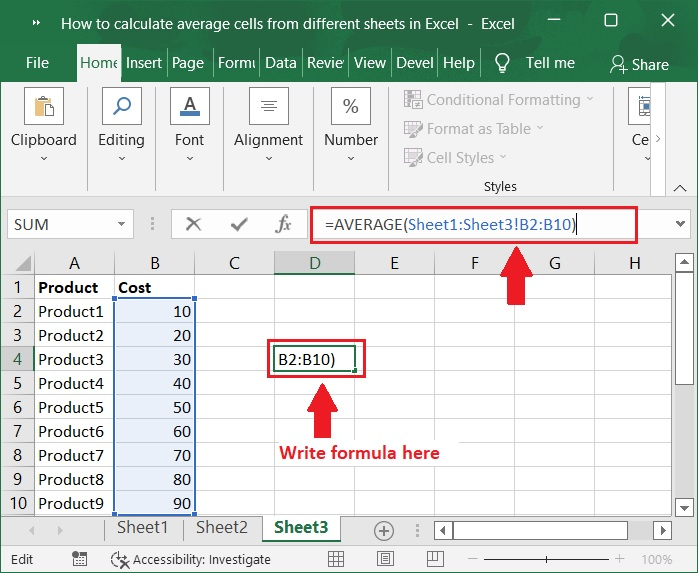### Step 3

Then press the Enter key. Now, you will receive the overall average in the cell D3. Please check out below screenshot for the same.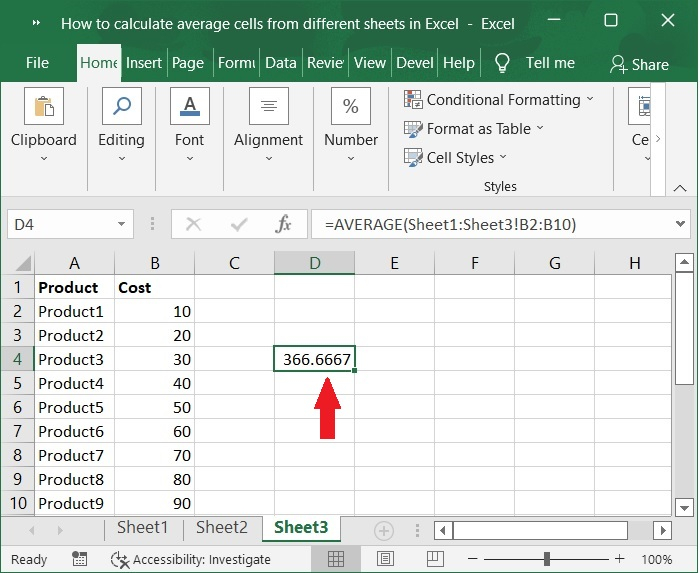### Important Note

• Sheet1 through Sheet3 are the many adjacent sheets from which you will compute the average using the formula "=AVERAGE(Sheet1:Sheet3!B1:B10)".

• The range B1 through B10 refers to the cells or range from which you will calculate the average in each of the multiple sheets. You are allowed to modify them to suit your requirements.

• If you want to calculate the average of multiple cells or ranges from multiple worksheets in Excel, for example, if you want to calculate the average of Range B1:B5 in Sheet1, Range B3:B6 in Sheet2, and Range B7:B9 in Sheet3, you should apply this formula: "=AVERAGE(B1:B5,Sheet2!B3:B6,Sheet3!7:B9)".

## Conclusion

In this tutorial, we used a simple example to demonstrate how you can calculate average cells from different sheets by using formulas in Excel.